## Faraday's law and Lenz's law

2-18-98

Relevant sections in the book : 22.4 - 22.5

We'll move from the qualitative investigation of induced emf to the quantitative picture. As we have learned, an emf can be induced in a coil if the magnetic flux through the coil is changed. It also makes a difference how fast the change is; a quick change induces more emf than a gradual change. This is summarized in Faraday's law of induction. The induced emf in a coil of N loops produced by a change in flux in a certain time interval is given by: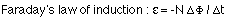Recalling that the flux through a loop of area A is given by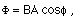The negative sign in Faraday's law comes from the fact that the emf induced in the coil acts to oppose any change in the magnetic flux. This is summarized in Lenz's law.

Lenz's law: The induced emf generates a current that sets up a magnetic field which acts to oppose the change in magnetic flux.

Another way of stating Lenz's law is to say that coils and loops like to maintain the status quo (i.e., they don't like change). If a coil has zero magnetic flux, when a magnet is brought close then, while the flux is changing, the coil will set up its own magnetic field that points opposite to the field from the magnet. On the other hand, a coil with a particular flux from an external magnetic field will set up its own magnetic field in an attempt to maintain the flux at a constant level if the external field (and therefore flux) is changed.

### An example

Consider a flat square coil with N = 5 loops. The coil is 20 cm on each side, and has a magnetic field of 0.3 T passing through it. The plane of the coil is perpendicular to the magnetic field: the field points out of the page.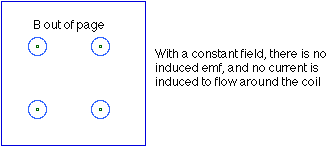(a) If nothing is changed, what is the induced emf?

There is only an induced emf when the magnetic flux changes, and while the change is taking place. If nothing changes, the induced emf is zero.

(b) The magnetic field is increased uniformly from 0.3 T to 0.8 T in 1.0 seconds. While the change is taking place, what is the induced emf in the coil?

Probably the most straight-forward way to approach this is to calculate the initial and final magnetic flux through the coil.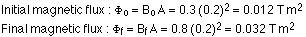The induced emf is then: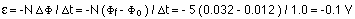(c) While the magnetic field is changing, the emf induced in the coil causes a current to flow. Does the current flow clockwise or counter-clockwise around the coil?

To answer this, apply Lenz's law, as well as the right-hand rule. While the magnetic field is being changed, the magnetic flux is being increased out of the page. According to Lenz's law, the emf induced in the loop by this changing flux produces a current that sets up a field opposing the change. The field set up by the current in the coil, then, points into the page, opposite to the direction of the increase in flux. To produce a field into the page, the current must flow clockwise around the loop. This can be found from the right hand rule.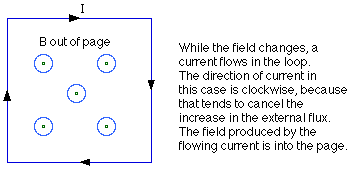One way to apply the rule is this. Point the thumb on your right hand in the direction of the required field, into the page in this case. If you curl your fingers, they curl in the direction the current flows around the loop - clockwise.